# ISEE Lower Level Math : How to find the area of a trapezoid

## Example Questions

2 Next →

### Example Question #11 : How To Find The Area Of A Trapezoid

Find the area of the trapezoid.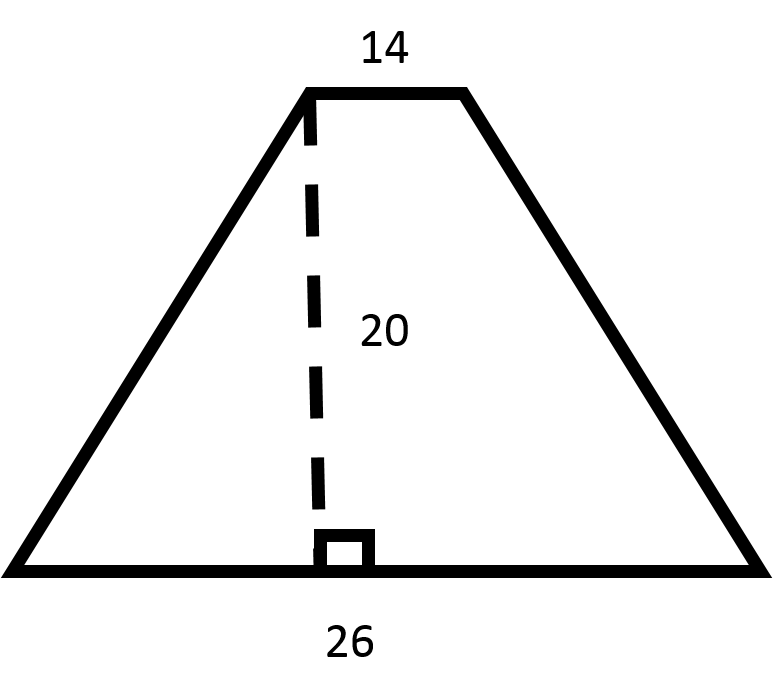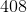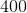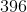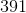Explanation:

Recall the formula for finding the area of a trapezoid: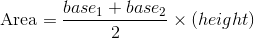Now, plug in the values for the bases and the height to find the area.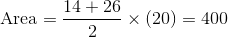### Example Question #282 : Geometry

Find the area of the trapezoid.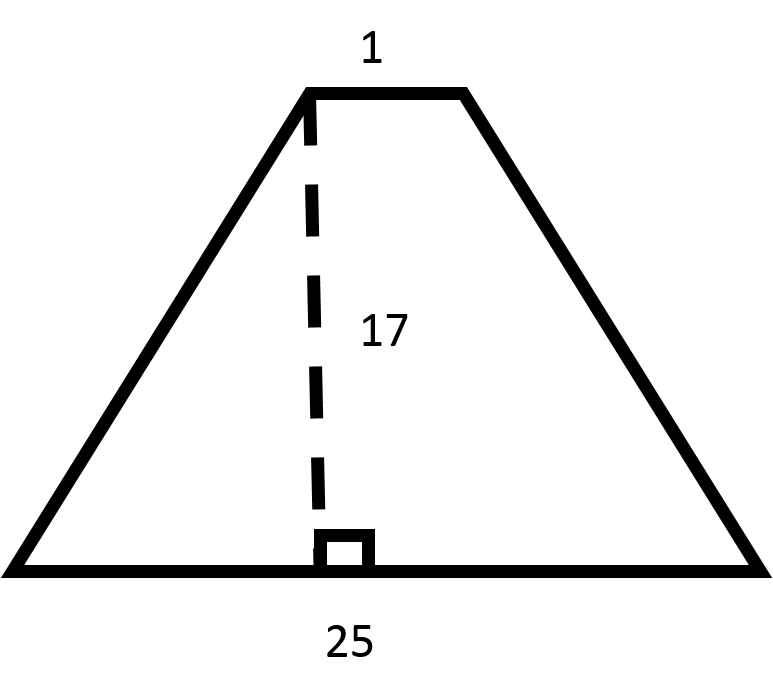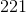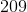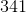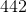Explanation:

Recall the formula for finding the area of a trapezoid:Now, plug in the values for the bases and the height to find the area.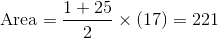### Example Question #283 : Geometry

Find the area of the trapezoid.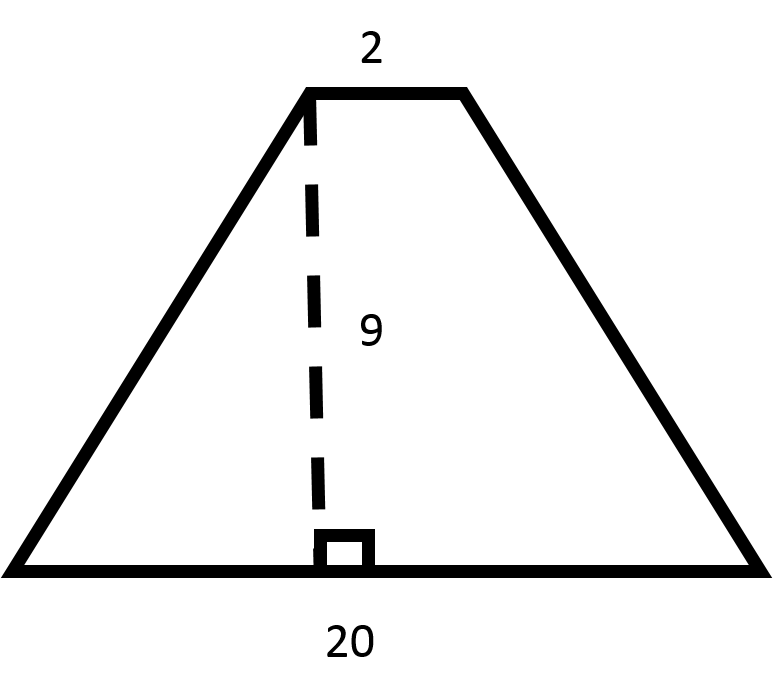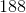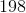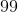Explanation:

Recall the formula for finding the area of a trapezoid:Now, plug in the values for the bases and the height to find the area.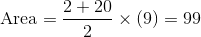### Example Question #284 : Geometry

Find the area of the trapezoid.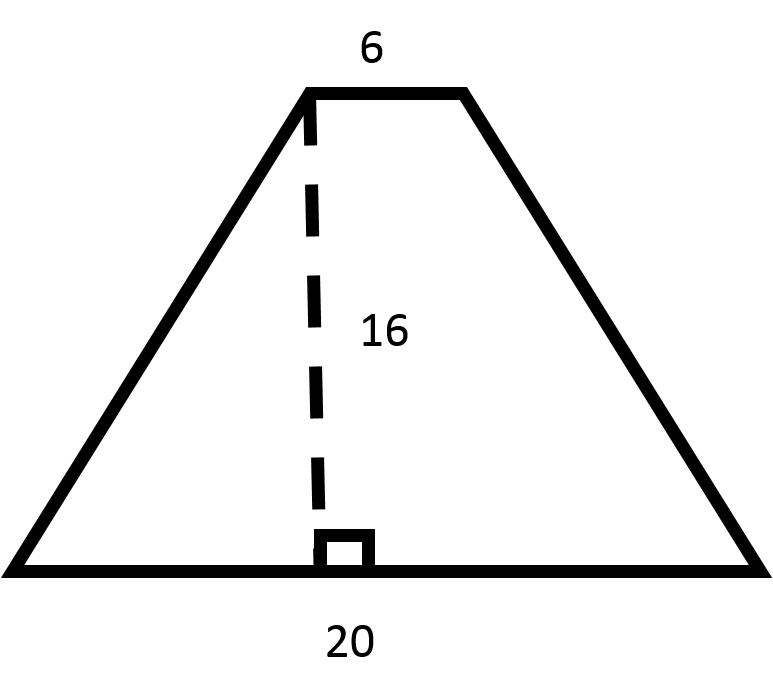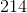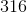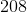Explanation:

Recall the formula for finding the area of a trapezoid:Now, plug in the values for the bases and the height to find the area.### Example Question #285 : Geometry

Find the area of the trapezoid.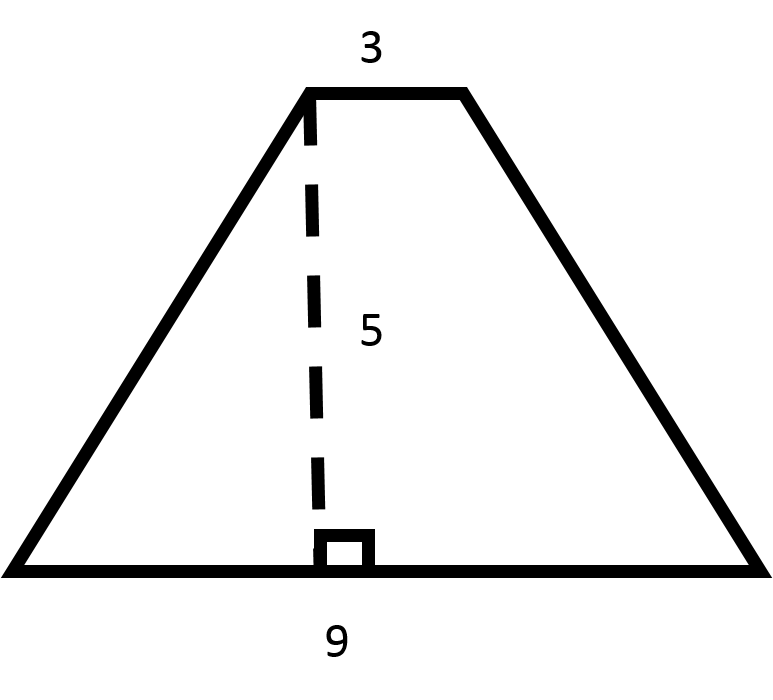Explanation:

Recall the formula for finding the area of a trapezoid:Now, plug in the values for the bases and the height to find the area.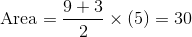### Example Question #286 : Geometry

Find the area of the trapezoid.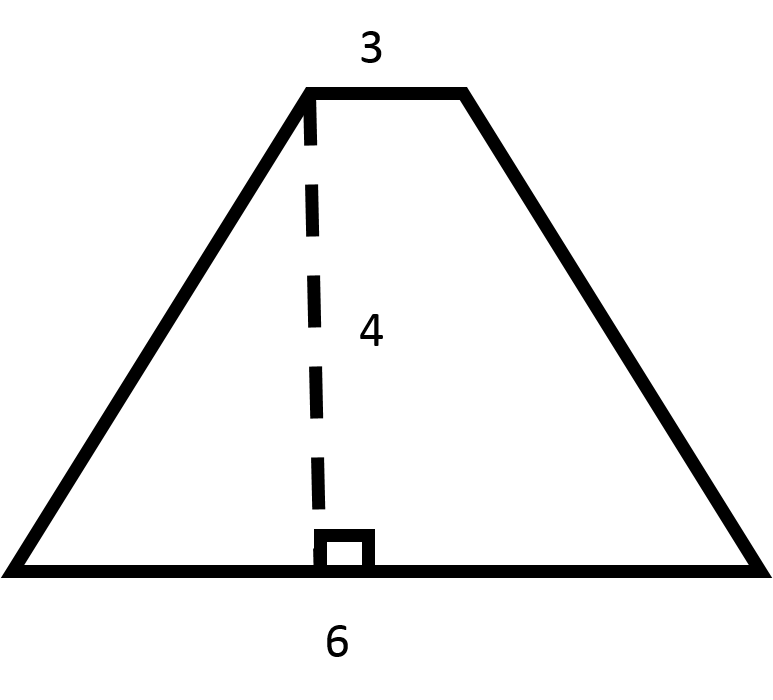Explanation:

Recall the formula for finding the area of a trapezoid:Now, plug in the values for the bases and the height to find the area.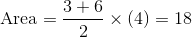### Example Question #287 : Geometry

Find the area of the trapezoid.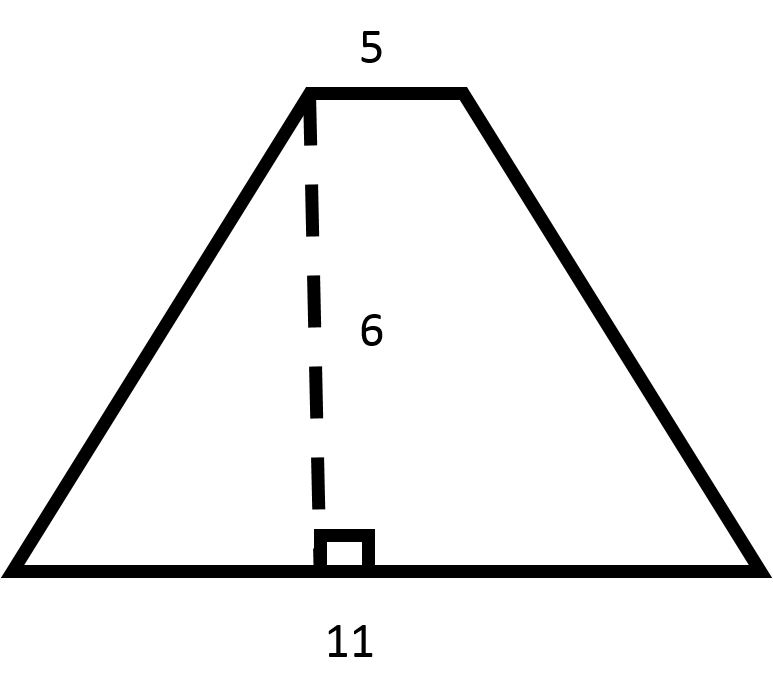Explanation:

Recall the formula for finding the area of a trapezoid:Now, plug in the values for the bases and the height to find the area.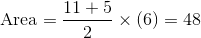### Example Question #288 : Geometry

Find the area of the trapezoid.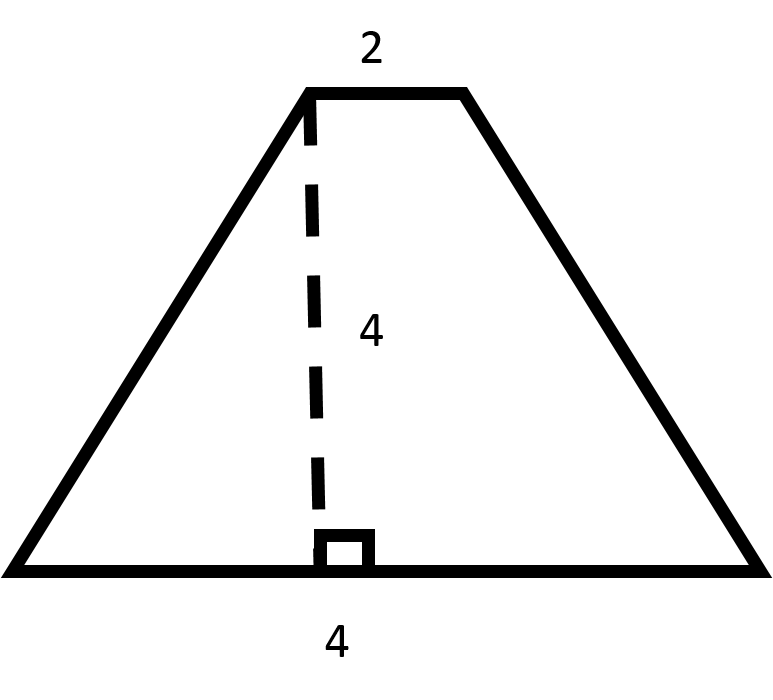Explanation:

Recall the formula for finding the area of a trapezoid:Now, plug in the values for the bases and the height to find the area.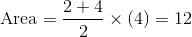### Example Question #289 : Geometry

Find the area of the trapezoid.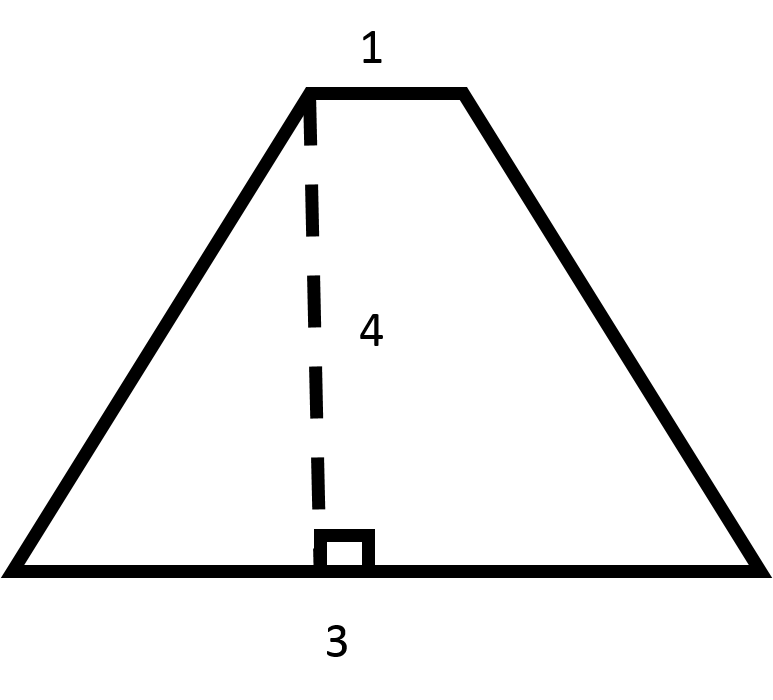Explanation:

Recall the formula for finding the area of a trapezoid:Now, plug in the values for the bases and the height to find the area.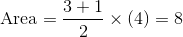### Example Question #290 : Geometry

Find the area of the trapezoid.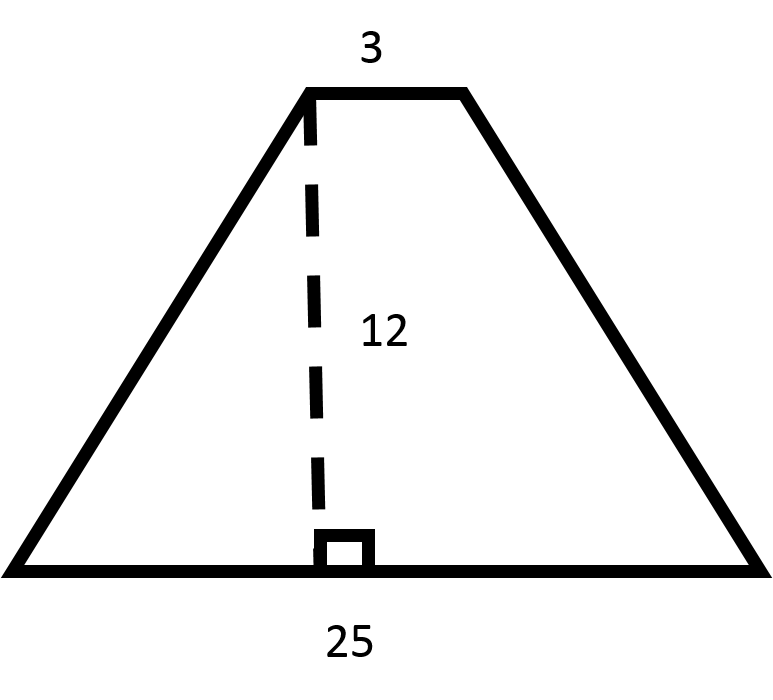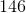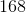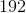Explanation:

Recall the formula for finding the area of a trapezoid:Now, plug in the values for the bases and the height to find the area.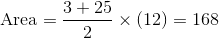2 Next →